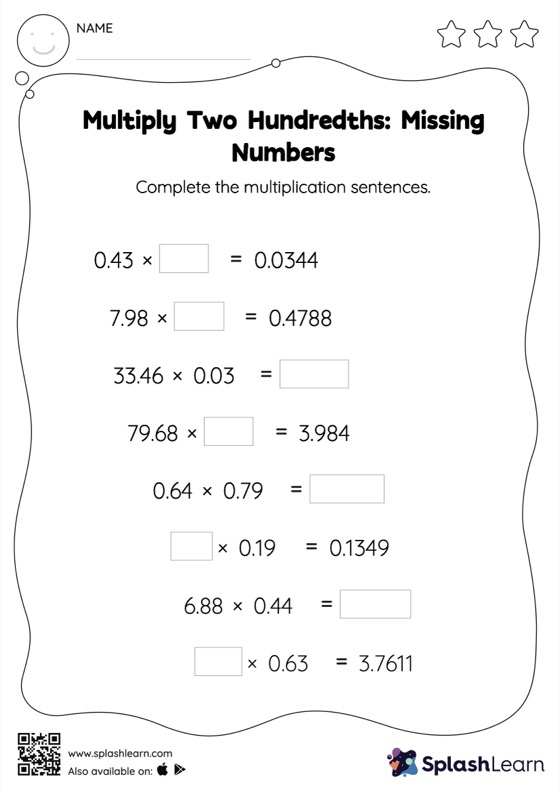# Multiply Two Hundredths: Missing Numbers Worksheet

Home > Multiply Two Hundredths: Missing NumbersGive your little one some practice building multiplication skills with this multiply two hundredths worksheet. When students multiply two decimals, they first multiply as if there is no decimal. They find the product and add the decimal point at the appropriate place in it. Students build on this idea to find the missing number in decimal multiplication in multiply two hundredths worksheet.